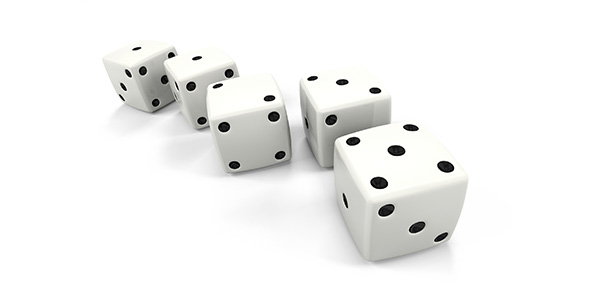# Probability And Odds Quiz Questions

10 Questions | Attempts: 1598
ShareSettingsThis quiz is all about probability and odds! Probability is the measure of the likelihood of which an event will occur. The higher a probability, the higher the odds are that the event will occur. Answer questions on probability below.

• 1.
For questions 1-6 please use the following information:  There are 20 marbles in a bag, 4 blue, 4 green, 4 red, 4 white and 4 black.  Remember that all fraction answers should be given in the simplest form.What is the probability of drawing a blue marble?
• A.

1/20

• B.

4/20

• C.

4/5

• D.

1/5

• 2.
What is the odds of drawing a red marble
• A.

1/3

• B.

3/1

• C.

4/15

• D.

1/5

• 3.
What is the probability of drawing a black or white marble?
• A.

2/3

• B.

8/12

• C.

8/20

• D.

2/5

• 4.
What is the odds of drawing a KU color?  (Hint for non fans:  that is red or blue).
• A.

8/20

• B.

2/3

• C.

8/12

• D.

2/5

• 5.
What is the shortcut to finding probability?
• A.

Success/total

• B.

Success/failure

• C.

Failure/success

• D.

Failure/total

• 6.
What is the shortcut for finding odds?
• A.

Success/total

• B.

Success/failure

• C.

Failure/success

• D.

Failure/total

• 7.
How can you change probability to odds?
• A.

Success/total+failure

• B.

Success/total-failure

• C.

Failure/total-success

• D.

Failure/total+success

• 8.
How can you change probability to odds?
• A.

Success/total+failure

• B.

Success/total-failure

• C.

Failure/total-success

• D.

Failure/total+success

• 9.
If you have a standard deck of cards (52 cards) what is the probability of drawing the Queen of Hearts?
• A.

1/51

• B.

51/1

• C.

52/1

• D.

1/52

• 10.
If you have a standard deck of cards what is the odds of drawing an seven?
• A.

4/52

• B.

1/13

• C.

4/48

• D.

1/12

## Related TopicsBack to top
×

Wait!
Here's an interesting quiz for you.